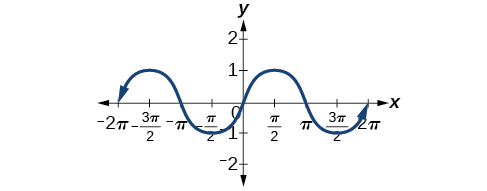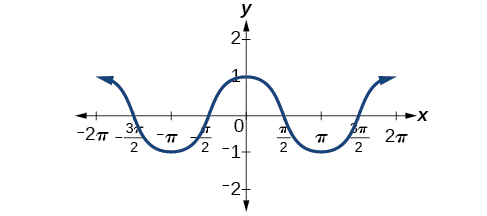# 8.1 Graphs of the sine and cosine functions  (Page 2/13)

 Page 2 / 13Odd symmetry of the sine function

[link] shows that the cosine function is symmetric about the y -axis. Again, we determined that the cosine function is an even function. Now we can see from the graph thatEven symmetry of the cosine function

## Characteristics of sine and cosine functions

The sine and cosine functions have several distinct characteristics:

• They are periodic functions with a period of $\text{\hspace{0.17em}}2\pi .$
• The domain of each function is $\text{\hspace{0.17em}}\left(-\infty ,\infty \right)\text{\hspace{0.17em}}$ and the range is $\text{\hspace{0.17em}}\left[-1,1\right].$
• The graph of is symmetric about the origin, because it is an odd function.
• The graph of is symmetric about the $\text{\hspace{0.17em}}y\text{-}$ axis, because it is an even function.

## Investigating sinusoidal functions

As we can see, sine and cosine functions have a regular period and range. If we watch ocean waves or ripples on a pond, we will see that they resemble the sine or cosine functions. However, they are not necessarily identical. Some are taller or longer than others. A function that has the same general shape as a sine or cosine function    is known as a sinusoidal function    . The general forms of sinusoidal functions are

## Determining the period of sinusoidal functions

Looking at the forms of sinusoidal functions, we can see that they are transformations of the sine and cosine functions. We can use what we know about transformations to determine the period.

In the general formula, $\text{\hspace{0.17em}}B\text{\hspace{0.17em}}$ is related to the period by $\text{\hspace{0.17em}}P=\frac{2\pi }{|B|}.\text{\hspace{0.17em}}$ If $\text{\hspace{0.17em}}|B|>1,\text{\hspace{0.17em}}$ then the period is less than $\text{\hspace{0.17em}}2\pi \text{\hspace{0.17em}}$ and the function undergoes a horizontal compression, whereas if $\text{\hspace{0.17em}}|B|<1,\text{\hspace{0.17em}}$ then the period is greater than $\text{\hspace{0.17em}}2\pi \text{\hspace{0.17em}}$ and the function undergoes a horizontal stretch. For example, $\text{\hspace{0.17em}}f\left(x\right)=\mathrm{sin}\left(x\right),\text{\hspace{0.17em}}$ $B=1,\text{\hspace{0.17em}}$ so the period is $\text{\hspace{0.17em}}2\pi ,\text{}$ which we knew. If $\text{\hspace{0.17em}}f\left(x\right)=\mathrm{sin}\left(2x\right),\text{\hspace{0.17em}}$ then $\text{\hspace{0.17em}}B=2,\text{\hspace{0.17em}}$ so the period is $\text{\hspace{0.17em}}\pi \text{\hspace{0.17em}}$ and the graph is compressed. If $\text{\hspace{0.17em}}f\left(x\right)=\mathrm{sin}\left(\frac{x}{2}\right),\text{\hspace{0.17em}}$ then $\text{\hspace{0.17em}}B=\frac{1}{2},\text{\hspace{0.17em}}$ so the period is $\text{\hspace{0.17em}}4\pi \text{\hspace{0.17em}}$ and the graph is stretched. Notice in [link] how the period is indirectly related to $\text{\hspace{0.17em}}|B|.$

## Period of sinusoidal functions

If we let $\text{\hspace{0.17em}}C=0\text{\hspace{0.17em}}$ and $\text{\hspace{0.17em}}D=0\text{\hspace{0.17em}}$ in the general form equations of the sine and cosine functions, we obtain the forms

$y=A\mathrm{sin}\left(Bx\right)$
$y=A\mathrm{cos}\left(Bx\right)$

The period is $\text{\hspace{0.17em}}\frac{2\pi }{|B|}.$

## Identifying the period of a sine or cosine function

Determine the period of the function $\text{\hspace{0.17em}}f\left(x\right)=\mathrm{sin}\left(\frac{\pi }{6}x\right).$

Let’s begin by comparing the equation to the general form $\text{\hspace{0.17em}}y=A\mathrm{sin}\left(Bx\right).$

In the given equation, $\text{\hspace{0.17em}}B=\frac{\pi }{6},\text{\hspace{0.17em}}$ so the period will be

Determine the period of the function $\text{\hspace{0.17em}}g\left(x\right)=\mathrm{cos}\left(\frac{x}{3}\right).$

$\text{\hspace{0.17em}}6\pi \text{\hspace{0.17em}}$

## Determining amplitude

Returning to the general formula for a sinusoidal function, we have analyzed how the variable $\text{\hspace{0.17em}}B\text{\hspace{0.17em}}$ relates to the period. Now let’s turn to the variable $\text{\hspace{0.17em}}A\text{\hspace{0.17em}}$ so we can analyze how it is related to the amplitude , or greatest distance from rest. $\text{\hspace{0.17em}}A\text{\hspace{0.17em}}$ represents the vertical stretch factor, and its absolute value $\text{\hspace{0.17em}}|A|\text{\hspace{0.17em}}$ is the amplitude. The local maxima will be a distance $\text{\hspace{0.17em}}|A|\text{\hspace{0.17em}}$ above the vertical midline of the graph, which is the line $\text{\hspace{0.17em}}x=D;\text{\hspace{0.17em}}$ because $\text{\hspace{0.17em}}D=0\text{\hspace{0.17em}}$ in this case, the midline is the x -axis. The local minima will be the same distance below the midline. If $\text{\hspace{0.17em}}|A|>1,\text{\hspace{0.17em}}$ the function is stretched. For example, the amplitude of $\text{\hspace{0.17em}}f\left(x\right)=4\text{\hspace{0.17em}}\mathrm{sin}\text{\hspace{0.17em}}x\text{\hspace{0.17em}}$ is twice the amplitude of $\text{\hspace{0.17em}}f\left(x\right)=2\text{\hspace{0.17em}}\mathrm{sin}\text{\hspace{0.17em}}x.\text{\hspace{0.17em}}$ If $\text{\hspace{0.17em}}|A|<1,\text{\hspace{0.17em}}$ the function is compressed. [link] compares several sine functions with different amplitudes.

#### Questions & Answers

A laser rangefinder is locked on a comet approaching Earth. The distance g(x), in kilometers, of the comet after x days, for x in the interval 0 to 30 days, is given by g(x)=250,000csc(π30x). Graph g(x) on the interval [0, 35]. Evaluate g(5)  and interpret the information. What is the minimum distance between the comet and Earth? When does this occur? To which constant in the equation does this correspond? Find and discuss the meaning of any vertical asymptotes.
Kaitlyn Reply
The sequence is {1,-1,1-1.....} has
amit Reply
circular region of radious
Kainat Reply
how can we solve this problem
Joel Reply
Sin(A+B) = sinBcosA+cosBsinA
Eseka Reply
Prove it
Eseka
Please prove it
Eseka
hi
Joel
June needs 45 gallons of punch. 2 different coolers. Bigger cooler is 5 times as large as smaller cooler. How many gallons in each cooler?
Arleathia Reply
7.5 and 37.5
Nando
find the sum of 28th term of the AP 3+10+17+---------
Prince Reply
I think you should say "28 terms" instead of "28th term"
Vedant
the 28th term is 175
Nando
192
Kenneth
if sequence sn is a such that sn>0 for all n and lim sn=0than prove that lim (s1 s2............ sn) ke hole power n =n
SANDESH Reply
write down the polynomial function with root 1/3,2,-3 with solution
Gift Reply
if A and B are subspaces of V prove that (A+B)/B=A/(A-B)
Pream Reply
write down the value of each of the following in surd form a)cos(-65°) b)sin(-180°)c)tan(225°)d)tan(135°)
Oroke Reply
Prove that (sinA/1-cosA - 1-cosA/sinA) (cosA/1-sinA - 1-sinA/cosA) = 4
kiruba Reply
what is the answer to dividing negative index
Morosi Reply
In a triangle ABC prove that. (b+c)cosA+(c+a)cosB+(a+b)cisC=a+b+c.
Shivam Reply
give me the waec 2019 questions
Aaron Reply

### Read also:

#### Get the best Algebra and trigonometry course in your pocket!

Source:  OpenStax, Algebra and trigonometry. OpenStax CNX. Nov 14, 2016 Download for free at https://legacy.cnx.org/content/col11758/1.6
Google Play and the Google Play logo are trademarks of Google Inc.

Notification Switch

Would you like to follow the 'Algebra and trigonometry' conversation and receive update notifications?ByByByBy RhodesBy